# AP Board 7th Class Maths Solutions Chapter 10 Construction of Triangles Review Exercise

SCERT AP 7th Class Maths Solutions Pdf Chapter 10 Construction of Triangles Review Exercise Questions and Answers.

## AP State Syllabus 7th Class Maths Solutions 10th Lesson Construction of Triangles Review Exercise

Question 1.
Draw the angles 70° and 110° by using a protractor,
(i) ∠AOB = 70°
∠AOB = 70°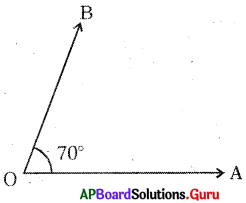1. Draw a ray $$\overrightarrow{\mathrm{OA}}$$
2. At ‘O’ erect a ray OB such that ∠AOB = 70°

(ii) ∠AOB = 110°
∠AOB = 110°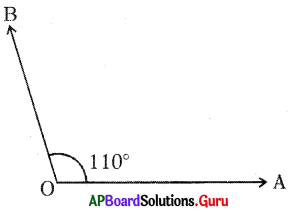1. Draw a ray $$\overrightarrow{\mathrm{OA}}$$.
2. At ‘O’ erect a ray $$\overrightarrow{\mathrm{OB}}$$ such that ∠AOB = 110°

Question 2.
Construct the angles 60° and 120° by using ruler and compass.
(i) ∠AOB = 60°
∠AOB = 60°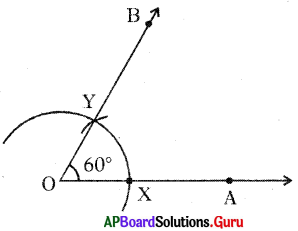1. Draw a ray $$\overrightarrow{\mathrm{OA}}$$.
2. Draw an arc with any radius (convenient) taking ‘O’ as a centre and cutting $$\overrightarrow{\mathrm{OA}}$$ at X.
3. Draw an arc with the same radius – taking X as a centre and meeting the previous arc at Y.
4. Join OY and produce it to B.
5. ∠AOB is formed.
6. ∠AOB = 60°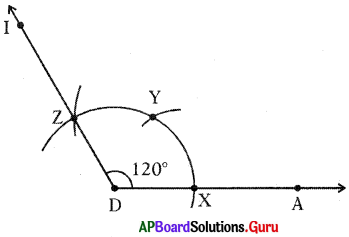1. Draw a ray $$\overrightarrow{\mathrm{DA}}$$.
2. Draw an arc with any radius taking ‘D’ as centre intersecting $$\overrightarrow{\mathrm{DA}}$$ at X.
3. Now draw two successive arcs from X intersecting the previous arc at Y and Z.
4. Join D and Z and produce DZ to I.
5. Now the angle formed ∠ADI is equal to 120°.Question 3.
Draw a line segment PQ = 4.5 cm and construct its perpendicular bisector by using ruler and compass.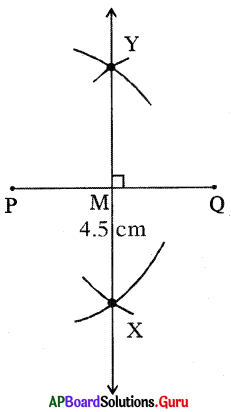∠PMY = ∠QMY = 90°
So, PQ ⊥ XY

1. Draw a line segment PQ of length 4.5 cm.
2. Draw two arcs with centre P and radius > $$\frac{1}{2}$$PQ on both sides of PQ.
3. Repeat step 2 with centre ‘Q’ intersecting the previous arc at X and Y.
4. Join X and Y and produce it on either sides to form $$\overrightarrow{\mathrm{XY}}$$.
5. XY is the perpendicular bisector of PQ.

Question 4.
Construct ∠DEF = 60° and its angular bisector by using ruler and compass.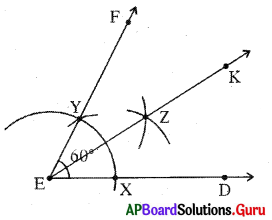∠DEF = 60°
∠DEK = ∠FEK = $$\frac{\angle \mathrm{DEF}}{2}=\frac{60^{\circ}}{2}$$ = 30°

1. Construct ∠DEF = 60°
2. Draw an arc with a convenient radius taking E as centre intersecting ED at X and EF at Y.
3. Draw two arcs with the same radius taking X and Y as centres intersecting at Z.
4. Join E, Z and produce it to K.
5. ∠DEK = ∠KEF = 30°.Question 5.
Construct an angle of 90° without using a protractor.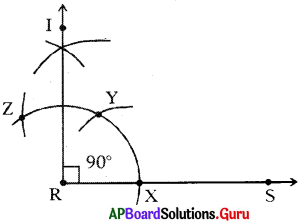1. Draw a ray $$\overrightarrow{R S}$$.
2. Draw an arc of convenient radius taking R as a centre and intersecting $$\overrightarrow{R S}$$ at X.
4. Draw bisector to $$\widehat{\mathrm{YZ}}$$ intersecting at I.